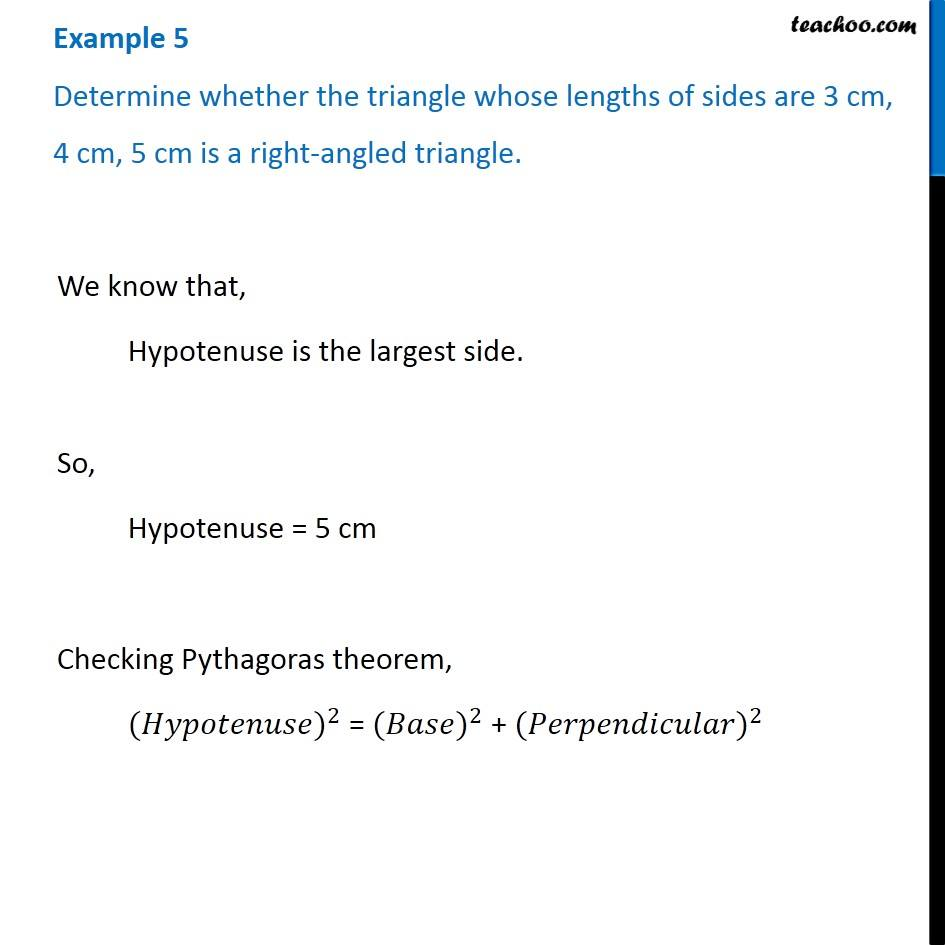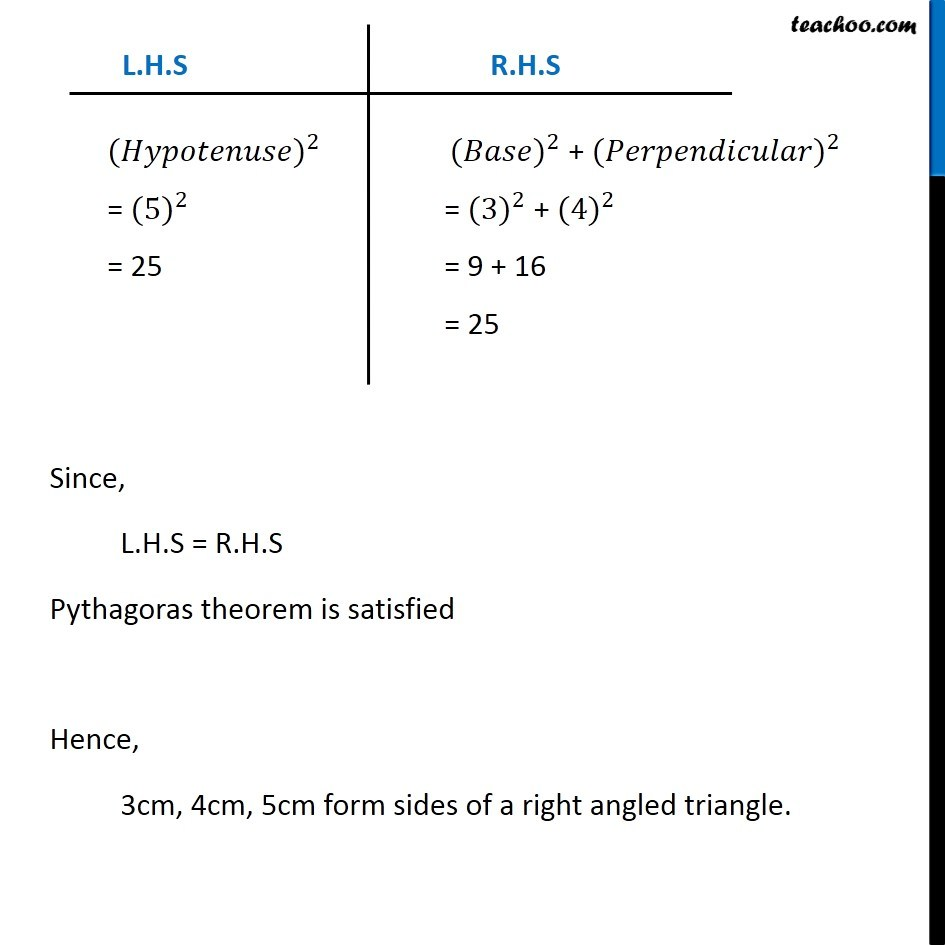Examples

Chapter 6 Class 7 Triangle and its Properties
Serial order wiseLearn in your speed, with individual attention - Teachoo Maths 1-on-1 Class

### Transcript

Example 5 Determine whether the triangle whose lengths of sides are 3 cm, 4 cm, 5 cm is a right-angled triangle. We know that, Hypotenuse is the largest side. So, Hypotenuse = 5 cm Checking Pythagoras theorem, 〖(𝐻𝑦𝑝𝑜𝑡𝑒𝑛𝑢𝑠𝑒)〗^2 = 〖(𝐵𝑎𝑠𝑒)〗^2 + 〖(𝑃𝑒𝑟𝑝𝑒𝑛𝑑𝑖𝑐𝑢𝑙𝑎𝑟)〗^2 〖(𝐻𝑦𝑝𝑜𝑡𝑒𝑛𝑢𝑠𝑒)〗^2 = 〖(5)〗^2 = 25 〖(𝐵𝑎𝑠𝑒)〗^2 " + " 〖(𝑃𝑒𝑟𝑝𝑒𝑛𝑑𝑖𝑐𝑢𝑙𝑎𝑟)〗^2 = 〖(3)〗^2 + 〖(4)〗^2 = 9 + 16 = 25 Since, L.H.S = R.H.S Pythagoras theorem is satisfied Hence, 3cm, 4cm, 5cm form sides of a right angled triangle. Since, L.H.S = R.H.S Pythagoras theorem is satisfied Hence, 3cm, 4cm, 5cm form sides of a right angled triangle. Since, L.H.S = R.H.S Pythagoras theorem is satisfied Hence, 3cm, 4cm, 5cm form sides of a right angled triangle.